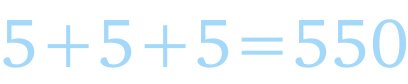Logic puzzles, riddles, math puzzles and brainteasers - pzzls.com

Vandaag is het 4 December 2023

Sort - Most popular on top
Page: << 1 2 3 4 5 >>

# Divisible from 1 to 9 - math puzzle

Difficulty:Rating: 3.6/5.0

Find a number consisting of 9 digits in which each of the digits from 1 to 9 appears only once. This number should satisfy the following requirements:
a. The number should be divisible by 9.
b. If the most right digit is removed, the remaining number should be divisible by 8.
c. If then again the most right digit is removed, the remaining number should be divisible by 7.
d. etc. until the last remaining number of one digit which should be divisible by 1.

# Lockers - math puzzle

Difficulty:Rating: 3.6/5.0

A high school has a strange principal. On the first day, he has his students perform an odd opening day ceremony:

There are one thousand lockers and one thousand students in the school. The principal asks the first student to go to every locker and open it. Then he has the second student go to every second locker and close it. The third goes to every third locker and, if it is closed, he opens it, and if it is open, he closes it. The fourth student does this to every fourth locker, and so on. After the process is completed with the thousandth student, how many lockers are open?

# Straight line sum - math puzzle

Difficulty:Rating: 3.3/5.0